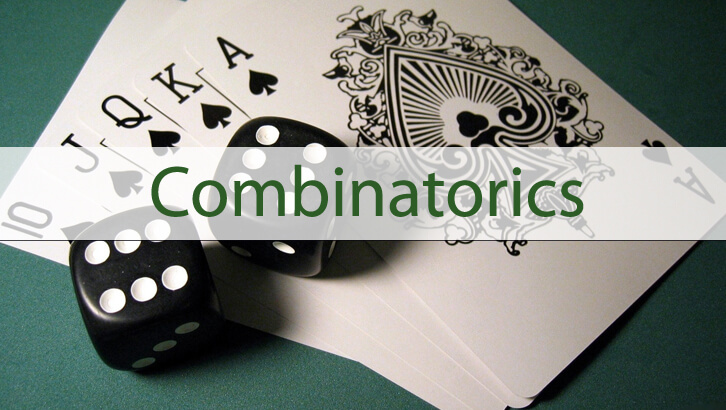CAT Permutation and Combinations

Question

If we list all the words that can be formed by rearranging the letters of the word SLEEPLESS in alphabetical order, what would be the rank of SLEEPLESS?

Explanation

First let us think about the number of possible rearrangements. SLEEPLESS can be rearranged in 9!/ (2! 3! 3!) = 5040.

Now, if rearrange these, we would have words starting with E, L, P and S.

Now, SLEEPLESS starts with S. So, let us think about how many words start with S. Number of words starting with S = 8!/ (2! 2! 3!) = 1680.

So, there would have been 5040 – 1680 words that have gone by before the first word starting with S. Or, 3360 words start with E, L or P.

The first word with S is SEEELLPSS. This would have a rank of 3361.

Now, let us go step by step.

Words starting with SE____ – Number of such words = 7!/(2!*2!*2!) = 630 words

Next we have words starting with SL, but our word also starts with SL, so let us go deeper

Words starting with SLE would be the next step, but our word starts with SLE as well.SO, let us go one further step deeper

Words starting with SLEE. The first such word would be SLEEEPLSS

So, let us think about words starting with SLEEE – there would be 4!/(2!) words like this = 12 words like this

words starting with SLEEL – there would be 4!/(2!) words like this = 12 words like this

So, we have accounted for 3360 + 630 + 12 + 12 = 4014 words thus far.

Now, on to words starting with SLEEP – there are again 4!/(2!) words like this = 12 words like this

Our word is within these 12 words

Words starting with SLEEPE – there are 3!/2! words like this, or 3 words like this
Our word comes in the next bunch.

Words starting with SLEEPL –  SLEEPLESS is the first such word. Or, the rank fo SLEEPLESS = 4014 + 3 + 1 = 4018.

A very good question to get lots of practice on letters rearrangement. However, it is unlikely that you will face a question this time-consuming in CAT. Wonderful question to practice though.